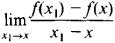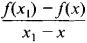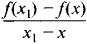derivative

(redirected from Derivative (mathematics))
Also found in: Dictionary, Thesaurus, Medical, Legal, Financial.

derivative:

see calculuscalculus,
branch of mathematics that studies continuously changing quantities. The calculus is characterized by the use of infinite processes, involving passage to a limit—the notion of tending toward, or approaching, an ultimate value.
.
The following article is from The Great Soviet Encyclopedia (1979). It might be outdated or ideologically biased.

Derivative

the fundamental concept of the differential calculus. It characterizes the rate of change of a function. The derivative is a function defined, for every x, as the limit of the ratioif the limit exists. A function whose derivative exists is said to be differentiable.

Every differentiable function is continuous. The opposite assertion, however, is false. There even exist continuous functions that are nowhere differentiable. The derivative of a function of a real variable may be nondifferentiable or even discontinuous. In the complex domain, on the other hand, the existence of the first derivative implies the existence of derivatives of all orders. Derivatives of functions of more than one variable (partial derivatives), the rules for obtaining derivatives, and various applications of derivatives are discussed in the article DIFFERENTIAL CALCULUS.

The theory of functions of a real variable deals, in particular, with the functional properties of the derivative and with various generalizations of the concept of the derivative. For example, a derivative that exists everywhere is a function of class one in the Baire classification. A derivative, even if it is discontinuous, takes on all intermediate values between its maximum and minimum. The most important generalizations of the concept of derivative follow.

Dini derivatives. The superior limit of the ratioas x1x, x1 > x, is called the right upper derivative of f Δd. The right lower λd and left upper Δs and lower λs derivatives are defined in an analogous manner. If Δd = λds = λs), f(x) has a right (left) derivative at the point x. The ordinary derivative exists if all four Dini derivatives are finite and equal. Dini derivatives were introduced by the Italian mathematician U. Dini in 1878. In 1915, N. N. Luzin proved that if all four Dini derivatives are finite on some set, then, apart from a null set, the function has an ordinary derivative everywhere on the set.

Approximate derivative. The approximate derivative was introduced by A. Ia. Khinchin in 1916. It is the limit of the ratioas x1, approaches x on the points of a set for which x is a density point.

derivative

[də′riv·əd·iv]
(chemistry)
A substance that is made from another substance.
(mathematics)
The slope of a graph y = ƒ(x) at a given point c ; more precisely, it is the limit as h approaches zero of ƒ(c + h) - ƒ(c) divided by h. Also known as differential coefficient; rate of change.
McGraw-Hill Dictionary of Scientific & Technical Terms, 6E, Copyright © 2003 by The McGraw-Hill Companies, Inc.

derivative

1. Chem a compound that is formed from, or can be regarded as formed from, a structurally related compound
2. Maths
a. the change of a function, f(x), with respect to an infinitesimally small change in the independent variable, x; the limit of [f(a + Δx)--f(a)]/Δx, at x = a, as the increment, Δx, tends to 0. Symbols: df(x)/dx, fʹ(x), Df(x)
b. the rate of change of one quantity with respect to another
3. Psychoanal an activity that represents the expression of hidden impulses and desires by channelling them into socially acceptable forms
Collins Discovery Encyclopedia, 1st edition © HarperCollins Publishers 2005
Site: Follow: Share:
Open / Close